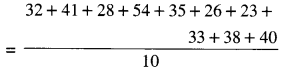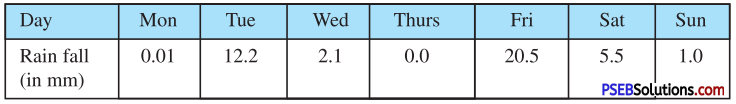# PSEB 7th Class Maths Solutions Chapter 3 Data Handling Ex 3.1

Punjab State Board PSEB 7th Class Maths Book Solutions Chapter 3 Data Handling Ex 3.1 Textbook Exercise Questions and Answers.

## PSEB Solutions for Class 7 Maths Chapter 3 Data Handling Ex 3.1

1. Find the mean of the following data :

Question (i).
3, 5, 7, 9, 11, 13, 15
Mean = $$\frac{3+5+7+9+11+13+15}{7}$$
= $$\frac {63}{7}$$
= 9

Question (ii).
40, 30, 30, 0, 26, 60
Mean = $$\frac{40+30+30+0+26+60}{6}$$
= $$\frac {183}{6}$$
= 9

2. Find the mean of the first five whole numbers.
The first five whole numbers are : 0, 1, 2, 3, 4
Mean = $$\frac{0+1+2+3+4}{5}$$
= $$\frac {10}{5}$$
= 2
Hence, the mean of first five whole numbers = 23. A batsman scored the following number of runs in six innings :
36, 35, 50, 46, 60, 55
Calculate the mean runs scored by him in an inning.
Mean runs
= $$\frac{36+35+50+46+60+55}{6}$$
= $$\frac {282}{6}$$
Hence, the mean runs scored by batsman in an innings = 47

4. The ages in years of 10 teachers of a school are :
32, 41, 28, 54, 35, 26, 23, 33, 38, 40
(i) What is the age of the oldest teacher and that of the youngest teacher ?
(ii) What is the range of the ages of the teachers ?
(iii) What is the mean age of these teachers ?
Arranging the ages in ascending order, we get
23, 26, 28, 32, 33, 35, 38, 40, 41, 54
(i) Age of the oldest teacher = 54 years Age of the youngest teacher 23 years
(ii) Range of the ages = 54 – 23 = 31
(iii) Mean age of the teachers= $$\frac {350}{10}$$
= 35 years.5. The rain fall (in mm) ip a city on 7 days of a certain week was recorded as follows :(i) Find the range of the rainfall in the above data.
(ii) Find the mean rainfall for the week.
(iii) How many days had the rainfall less than the mean rainfall ?
= $$\frac{0.01+12.2+2.1+0.0+20.5+5.5+1.0}{7}$$
= $$\frac {41.31}{7}$$